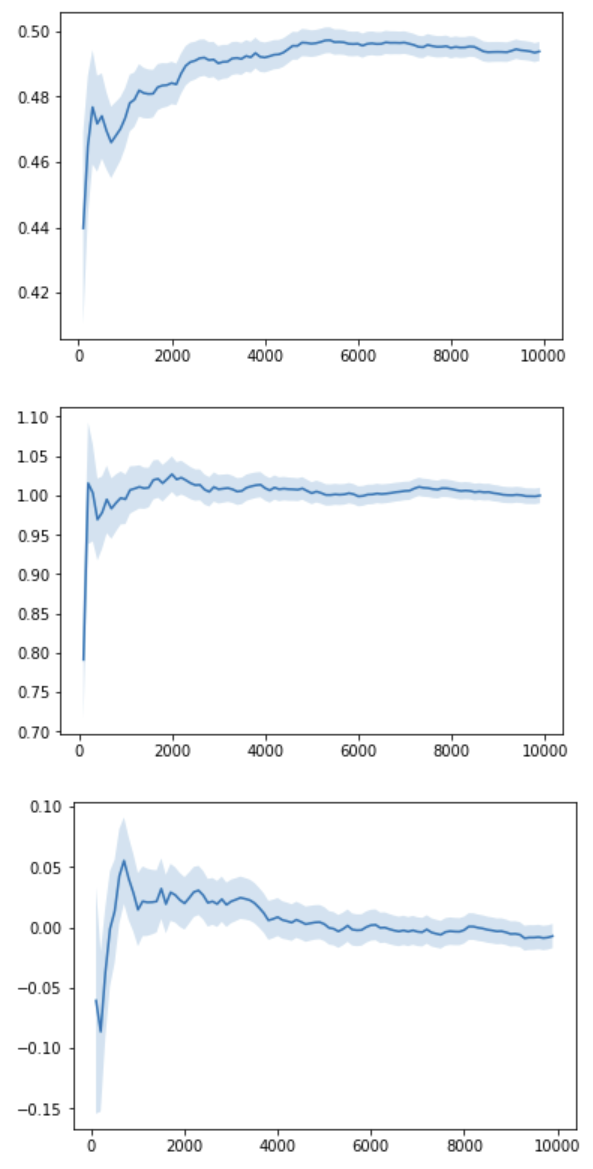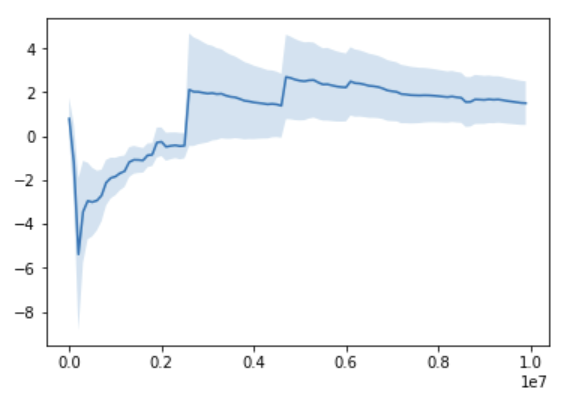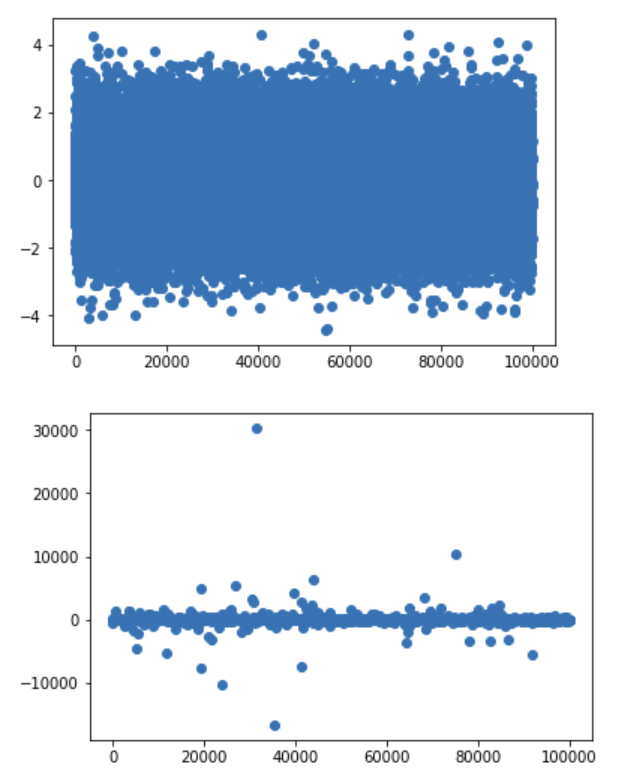# Beyond the Central Limit Theorem

Marton Trencseni - Thu 06 February 2020 - ab-testing

## Introduction

In the previous post, A/B testing and the Central Limit Theorem, I talked about the importance of the Central Limit Theorem (CLT) to A/B testing. Here we will explore cases when we cannot rely on the CLT to hold. Exploring when a theorem doesn’t hold is a good way to deepen our understanding why the theorem works when it works. It’s a bit like writing tests for software and trying to break it.

I will show 3 cases when we cannot rely on the CLT to hold:

1. the distribution does not have a mean, eg. the Cauchy distribution
2. violating the independence assumption of the CLT, eg. with a random walk
3. small sample size, eg. when events such as fraudulent transactions have a very low probability

The code shown below is up on Github.

## 1. The distribution does not have a mean

The CLT says that when we are approximating the mean of a distribution by sampling, the sample means follow a normal distribution. So the CLT is about approximating the mean of a distribution. What if the distribution does not have a mean? In cases like this, we can still sample it, and compute a mean, but:

• since the original distribution doesn't have a mean, we're not approximating it
• the sampled means will not converge to any value, they will keep jumping around

How is it even possible for a distribution not to have a mean? The mean, or expected value $E[X]$ of a continuous random variable $X$ with probability density function (pdf) $f$ is given by:

$E[X] = \int x f(x) dx$, where $\int f(x) dx = 1$

For a discrete random variable:

$E[X] = \sum i p_i$, where $\sum p_i = 1$

The mean of a distribution does not exist if the integral or sum does not exist, ie. for a "pathological" $f$ or $p_i$.

One example is the Cauchy-distribution, defined by $f(x) = \frac{ 1 }{ \pi ( 1 + x^2 )}$. If you plug this into the above integral, it does not exist.

The Cauchy distribution looks like this (shown with the normal in blue, cauchy in orange; notice how cauchy is more narrow in the center and fatter in the tails than the normal):We can see this for ourselves with a Monte Carlo simulation:

1. Draw samples from a distribution
2. Every 100 sample, compute the running mean (from the very beginning)
3. Plot the running mean, together with the standard error

Like in the previous post, we'll use scipy. First let's do this for distributions that have a mean: uniform ($\mu = 0.5$), exponential ($\mu = 1$) and a standard normal ($\mu = 0$):

def population_running_mean_plot(population, sample_size=10*1000):
sample = population.rvs(size=sample_size)
step_size = int(len(sample)/100)
running_stats = []
for i in range(100, len(sample), step_size):
running_sample = sample[:i]
running_stats.append(([i, mean(running_sample), sem(running_sample)]))
x = [x for x in running_stats]
y = [x for x in running_stats]
envelope_min = [x - x for x in running_stats]
envelope_max = [x + x for x in running_stats]
plt.plot(x, y)
plt.fill_between(x, envelope_min, envelope_max, alpha=0.2)
plt.show()
population_running_mean_plot(uniform)
population_running_mean_plot(expon)
population_running_mean_plot(norm)


Let's see how the running mean converges to the true sample mean after $N=10000$ samples:Let's do the same for the Cauchy distribution, but let's let it run for $N=10000000$ samples:For the Cauchy even after millions of samples, sometimes there is a big jump in the running mean (unlike the previous distributions). It does not converge: if we keep running it, it will keep jumping around, and it will jump to arbitrarily large values.

Why is this? The Cauchy distribution can be visualized like this: imagine drawing the bottom part of a circle (a half-circle) around the center (x=0, y=1). Each time we want to sample a number from the Cauchy distribution, first we pick an angle $\theta \in ( -\pi/2, \pi/2)$ in a uniform way on the half circle, and then shoot a ray from the center at that $\theta$ angle to the half-circle, and then on to the x-axis. The coordinate of the x-axis intercept is the returned value for the Cauchy sampling. Although the angle $\theta$ is uniform, the ray can shoot arbitrarily far on the x-axis to generate extremely large or small values, and this happens quite often (the fat tail). Note that the normal distribution can also generate arbitrarily small or large values, but at a lower rate, so the mean still exists there.

Another way to see this is to look at a histogram of $N=100000$ draws and compare it with a normal distribution (top is normal, bottom is Cauchy):The normal doesn't produce extreme values, whereas the Cauchy does.

## 2. Violating the independence assumption of the CLT

The wikipedia quote for the CLT starts like this: “...when independent random variables are added...”. In other words, the samples we draw should be independent of each other. What does this mean for an A/B test? For example, if user X and user Y are both using the product, they should not talk to each other, they should not influence each other when making the conversion “decision”, or when "deciding" how much time to spend with the product.

## Conclusion

There are a number of caveats when running A/B tests in real life. Making sure the CLT holds when we use a test statistic that assumes a normal distribution is one of them. From the list of considerations above, the top thing to keep in mind is the concern of sample sizes (#3). In SaaS-like environments, the population distribution will always exist (#1), especially if we winsorize our results (winsorization just means we replace the most extreme values at the two ends of our sample with less extreme values). Independence (#2) usually holds in SaaS environments, but may not in social networks.

It's worth remembering that there are also non-statistical A/B testing errors, eg. if we run variant A in Germany and variant B in France. We may get enough samples for both A and B, both are normals, we get a nice reading on the difference, but we're comparing apples to oranges. We're not measuring (just) the difference between A and B, but the difference between german and french users.